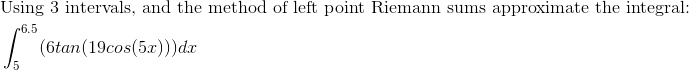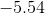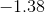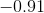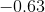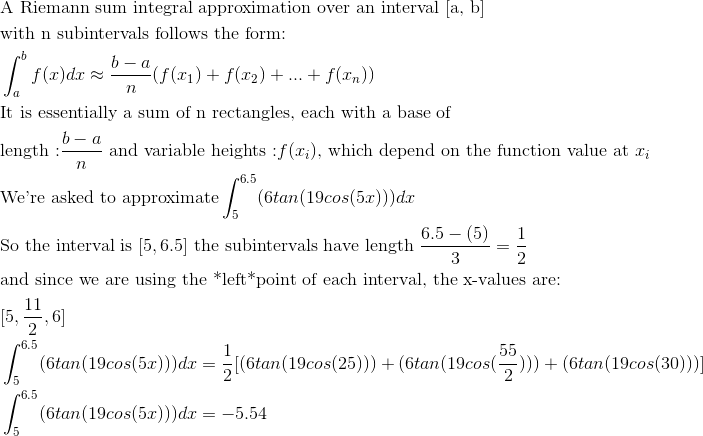AP Calculus BC : Riemann Sum: Left Evaluation

Example Questions

2 Next →

Example Question #11 : Riemann Sum: Left Evaluation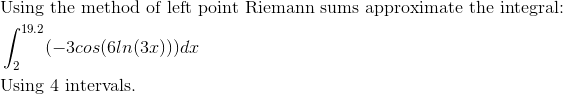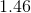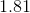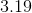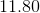Explanation: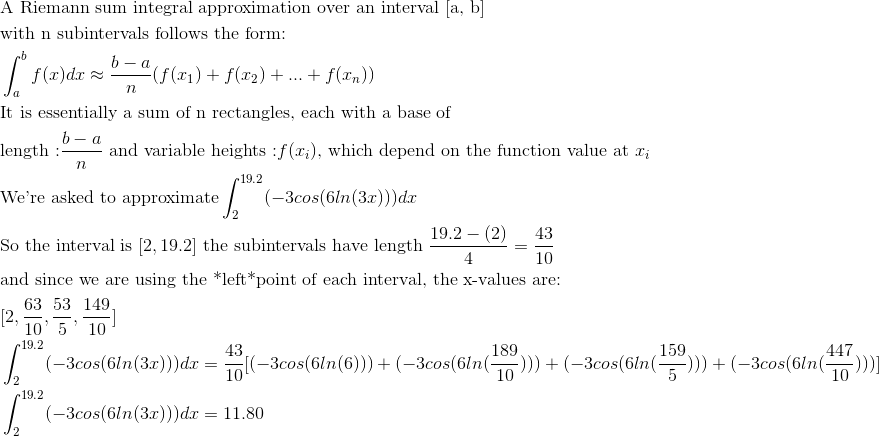Example Question #12 : Riemann Sum: Left Evaluation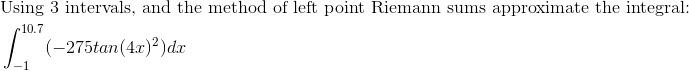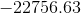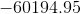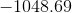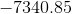Explanation: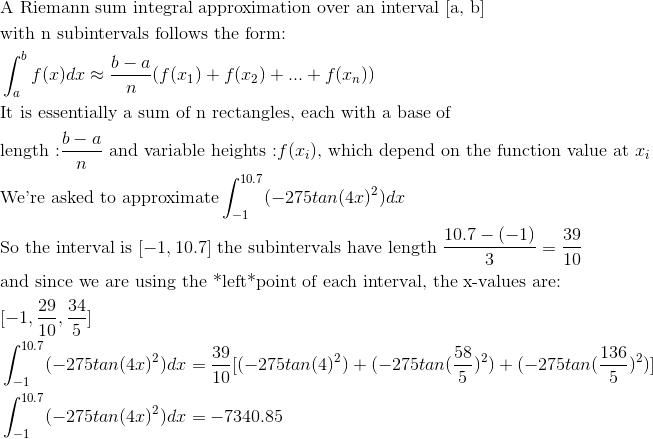Example Question #13 : Riemann Sum: Left Evaluation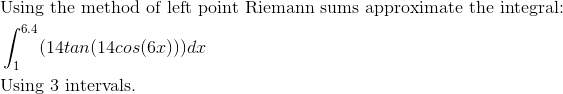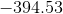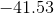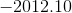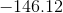Explanation: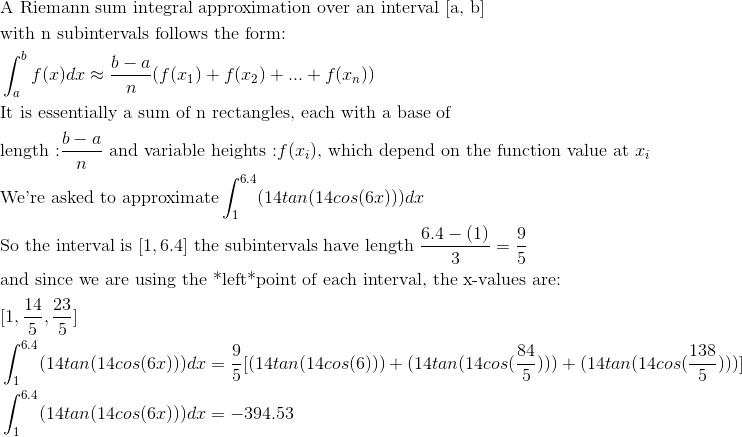Example Question #14 : Riemann Sum: Left Evaluation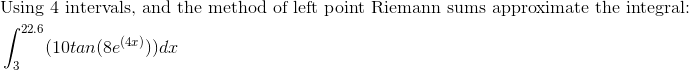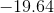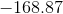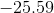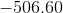Explanation: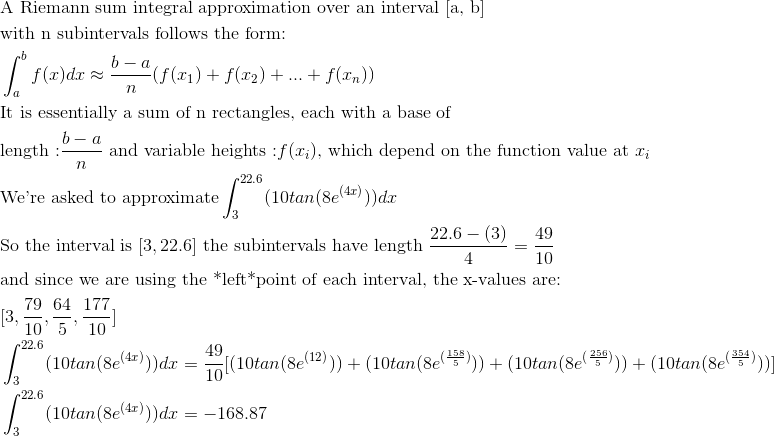Example Question #15 : Riemann Sum: Left Evaluation